# ML Aggarwal Class 7 Solutions for ICSE Maths Chapter 3 Rational Numbers Objective Type Questions

## ML Aggarwal Class 7 Solutions for ICSE Maths Chapter 3 Rational Numbers Objective Type Questions

Mental Maths

Question 1.
Fill in the blanks:
(i) Two rational numbers are called equivalent if they have …… value.
(ii) The number $$\frac { -4 }{ 7 }$$ lies to the …….. of zero on the number line.
(iii) The rational number $$\frac { -5 }{ -11 }$$ lies to the ……. of zero on the number line.
(iv) The rational number $$\frac { -84 }{ 156 }$$ reduced to simplest form is ………
(v) The standard form of the rational number $$\frac { 14 }{ -12 }$$ is ………
(vi) There are ….. rational numbers between two different rational numbers.
(vii) Two rational numbers $$\frac { p }{ q }$$and $$\frac { r }{ s }$$ are equal if an only if q × r = ……..
(viii)The multiplicative inverse of -3$$\frac { 1 }{ 5 }$$ is ……..
(ix) $$\frac { -3 }{ 7 }$$ ÷ $$\frac { -7 }{ 3 }$$ = ………
(x) If p and q are positive integers, then $$\frac { p }{ q }$$ is a …… rational number and $$\frac { p }{ -q }$$ is a ……
Solution: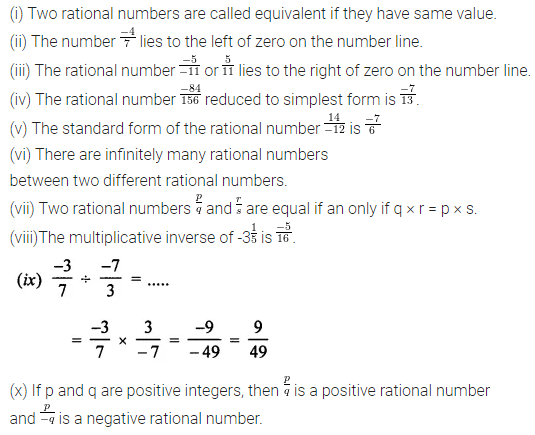Question 2.
State whether the following statements are true (T) or false (F).
(i) Zero is the smallest rational number.
(ii) Every integer is a rational number.
(iii) Every rational number is an integer.
(iv) Every fraction is a rational number.
(v) Every rational number is a fraction.
(vi) The reciprocal of -1 is -1.
(vii) The quotient of two integers is always a rational number.
(viii) The value of a rational number remains the same if both its numerator and denominator are multiplied (or divided) by the same (nonzero) integer.
(ix) Between two distinct integers, we can always insert an integer.
(x) Between two distinct rational numbers, we can al ways insert a rational number.
(xi) There exists atleast one integer between two different rational numbers.
(xii) The reciprocal of 2$$\frac { 6 }{ 7 }$$ is $$\frac { -7}{ 20 }$$.
(xiii) All terminating decimal numbers are rational numbers.
Solution: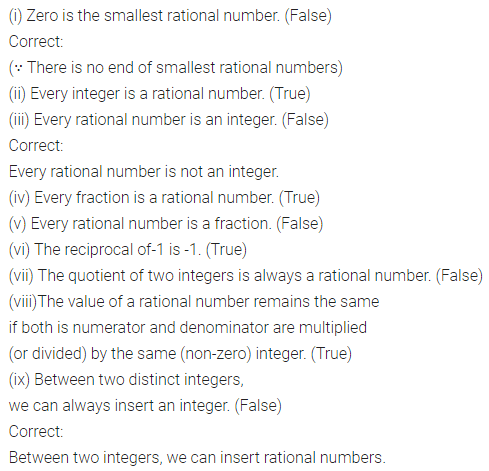Multiple Choice Questions

Choose the correct answer from the given four options (3 to 14):
Question 3.
The rational number $$\frac { 110 }{ -132 }$$ when reduced to standard form is
(a) $$\frac { 10 }{ -12 }$$
(b) $$\frac { 5 }{ -6 }$$
(c) $$\frac { -5 }{ 6 }$$
(d) $$\frac { 110 }{ -132 }$$
Solution: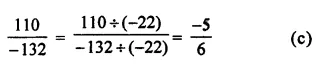Question 4.
The Additive inverse of $$\frac { -7 }{ 12 }$$ is
(a) $$\frac { 12 }{ -7 }$$
(b) $$\frac { -7 }{ 12 }$$
(c) $$\frac { -5 }{ 12 }$$
(d) $$\frac { 7 }{ 12 }$$
Solution: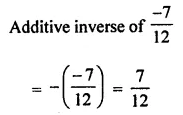Question 5.
The Multiplicative inverse of $$\frac { -4 }{ 9 }$$ is
(a) $$\frac { 4 }{ 9 }$$
(b) $$\frac { -9 }{ 4 }$$
(c) $$\frac { 9 }{ 4 }$$
(d) none of these
Solution: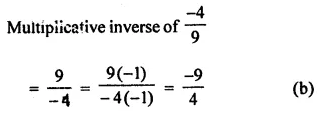Question 6.
The reciprocal of the rational number -2$$\frac { 3 }{ 7 }$$ is
(a) $$\frac { -17 }{ 7 }$$
(b) $$\frac { 7 }{ 17 }$$
(c) $$\frac { -7 }{ 17 }$$
(d) none of these
Solution: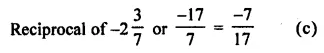Question 7.
The product of rational number $$\frac { -2 }{ 5 }$$ and its multiplicative inverse is
(a) 1
(b) 0
(c) $$\frac { 4 }{ 25 }$$
(d) $$\frac { 2 }{ 5 }$$
Solution: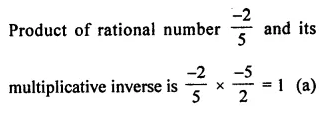Question 8.
The product of rational number $$\frac { -2 }{ 3 }$$ and its additive inverse is
(a) 1
(b) $$\frac { 2 }{ 3 }$$
(c) $$\frac { 4 }{ 9 }$$
(d) $$\frac { -4 }{ 9 }$$
Solution: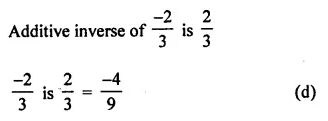Question 9.
The sum of rational number $$\frac { -1 }{ 3 }$$ and its reciprocal is
(a) 0
(b) 1
(c) $$\frac { -10 }{ 3 }$$
(d) $$\frac { -3 }{ 10 }$$
Solution: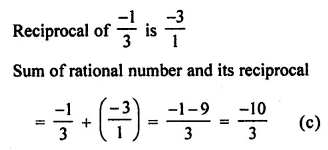Question 10.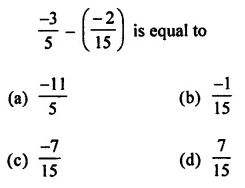Solution: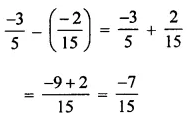Question 11.Solution: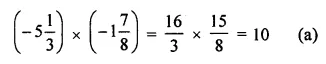Question 12.Solution: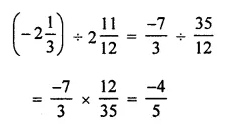Question 13.
In the standard form of a rational number, the denominator is always
(a) 0
(b) a negative integer
(c) 1
(d) a positive integer
Solution:Question 14.
The sum of two rational numbers is -1. If one of them is $$\frac { -5 }{ 7 }$$, then the other is
(a) $$\frac { 5 }{ 7 }$$
(b) $$\frac { -2 }{ 7 }$$
(c) $$\frac { 12 }{ 7 }$$
(d) $$\frac { -12 }{ 7 }$$
Solution: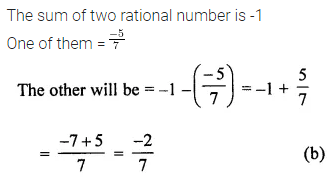Value Based Questions

Question 1.
Rohit donated $$\frac { 1 }{ 5 }$$ of his monthly income to an NGO working for the education of old women, $$\frac { 1 }{ 4 }$$ of his salary spent on food, $$\frac { 1 }{ 3 }$$ on rent and $$\frac { 1 }{ 15 }$$ on other expenses. If he is left with ₹ 9000, find his monthly income. What values are being promoted?
Solution: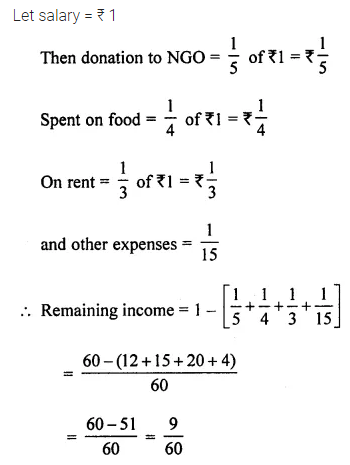Higher Order Thinking Skills (HOTS)

Question 1.
From a rope 15 m long, 4$$\frac { 1 }{ 3 }$$ m is cut off and $$\frac { 3 }{ 5 }$$ of the remaining is cut off again. Find the length of the remaining part of the rope.
Solution: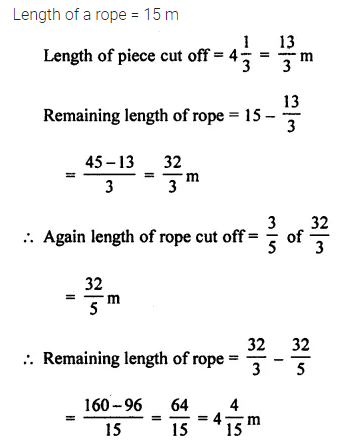Question 2.
Perimeter of a rectangle is 2 m less than $$\frac { 2 }{ 5 }$$ of the perimeter of a square, if the perimeter of the square is 40 m, find the length and breadth of the rectangle given that the breadth is $$\frac { 1 }{ 3 }$$ of the length.
Solution: